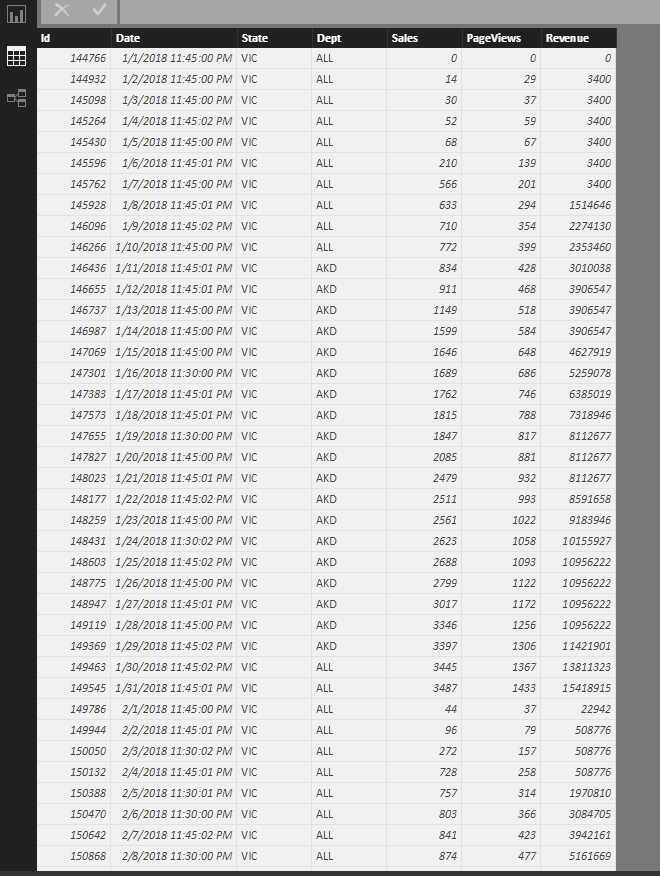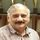cancel
Showing results for
Did you mean:

Fabric is Generally Available. Browse Fabric Presentations. Work towards your Fabric certification with the Cloud Skills Challenge.Helper V

## Calculating daily numbers based on MTD numbers

Hello There,

I'm working on a dataset which has MTD values but my requirement is to retrieve the values daily which is opposite of Running Total.

When I select the date range, I need cummulative sum between these respective days.

Regards,

Kalyan..1 ACCEPTED SOLUTIONCommunity Champion

Hi  @kkalyanrr,

Based on the data and your requirement, the approach to be taken is finding the previous days value and substracting it from the current days MTD value.

Try the following steps

1. I named the data table as Table2

2. Created an Indexcolumn from 1 in the fact table.

3. Created an YearMonth column as

YearMonth = Year(Table2[Date]) * 100 + Month(Table2[Date])

4.  Created a measure called DailySales

DailySales =
var PreviousValue =
CALCULATE(
SUM( Table2[Sales(MTD)] ),
FILTER(  ALL(Table2) ,
SUMX( FILTER( Table2, EARLIER(Table2[Index]) = Table2[Index] -1  && EARLIER([YearMonth]) = [YearMonth]  ),Table2[Sales(MTD)])
)
)

Return
Calculate(Sum(Table2[Sales(MTD)]) - PreviousValue)

In this measure we find the previousvalue first for each row and then finally substract ir from Sales[MTD]. This avoids creating a calculated column for the PreviousValue.

5.  Created measures DailyRevenue

DailyRevenue =
var PreviousValue =
CALCULATE(
SUM( Table2[Revenue(MTD)] ),
FILTER(  ALL(Table2) ,
SUMX( FILTER( Table2, EARLIER(Table2[Index]) = Table2[Index] -1  && EARLIER([YearMonth]) = [YearMonth]  ),Table2[Revenue(MTD)])
)
)

Return
Calculate(Sum(Table2[Revenue(MTD)]) - PreviousValue)

6. Created measure DailyPageViews

DailyPageViews =
var PreviousValue =
CALCULATE(
SUM( Table2[PageViews(MTD)] ),
FILTER(  ALL(Table2) ,
SUMX( FILTER( Table2, EARLIER(Table2[Index]) = Table2[Index] -1  && EARLIER([YearMonth]) = [YearMonth]  ),Table2[PageViews(MTD)])
)
)

Return
Calculate(Sum(Table2[PageViews(MTD)]) - PreviousValue)

Sample outputIf this works for you please accept it as a solution also give KUDOS.

Cheers

CheenuSing

Did I answer your question? Mark my post as a solution and also give KUDOS !

Proud to be a Datanaut!
6 REPLIES 6Community Champion

Can you share some data in the one drive or google drive and post the link here.  Also share the output expected from the dataset shared.

Cheers

CheenuSIng

Did I answer your question? Mark my post as a solution and also give KUDOS !

Proud to be a Datanaut!Helper VCommunity Champion

Hi  @kkalyanrr,

Based on the data and your requirement, the approach to be taken is finding the previous days value and substracting it from the current days MTD value.

Try the following steps

1. I named the data table as Table2

2. Created an Indexcolumn from 1 in the fact table.

3. Created an YearMonth column as

YearMonth = Year(Table2[Date]) * 100 + Month(Table2[Date])

4.  Created a measure called DailySales

DailySales =
var PreviousValue =
CALCULATE(
SUM( Table2[Sales(MTD)] ),
FILTER(  ALL(Table2) ,
SUMX( FILTER( Table2, EARLIER(Table2[Index]) = Table2[Index] -1  && EARLIER([YearMonth]) = [YearMonth]  ),Table2[Sales(MTD)])
)
)

Return
Calculate(Sum(Table2[Sales(MTD)]) - PreviousValue)

In this measure we find the previousvalue first for each row and then finally substract ir from Sales[MTD]. This avoids creating a calculated column for the PreviousValue.

5.  Created measures DailyRevenue

DailyRevenue =
var PreviousValue =
CALCULATE(
SUM( Table2[Revenue(MTD)] ),
FILTER(  ALL(Table2) ,
SUMX( FILTER( Table2, EARLIER(Table2[Index]) = Table2[Index] -1  && EARLIER([YearMonth]) = [YearMonth]  ),Table2[Revenue(MTD)])
)
)

Return
Calculate(Sum(Table2[Revenue(MTD)]) - PreviousValue)

6. Created measure DailyPageViews

DailyPageViews =
var PreviousValue =
CALCULATE(
SUM( Table2[PageViews(MTD)] ),
FILTER(  ALL(Table2) ,
SUMX( FILTER( Table2, EARLIER(Table2[Index]) = Table2[Index] -1  && EARLIER([YearMonth]) = [YearMonth]  ),Table2[PageViews(MTD)])
)
)

Return
Calculate(Sum(Table2[PageViews(MTD)]) - PreviousValue)

Sample outputIf this works for you please accept it as a solution also give KUDOS.

Cheers

CheenuSing

Did I answer your question? Mark my post as a solution and also give KUDOS !

Proud to be a Datanaut!Helper V

Thank you, Brilliant Stuff.Microsoft

Hi @kkalyanrr,

Please try this measure to get the cummulative sum

```Running total =
CALCULATE (
SUM ( 'Table'[Sales] ),
FILTER ( ALLSELECTED ( 'Table' ), 'Table'[Date] <= MAX ( 'Table'[Date] ) )
)```Best regards,

Yuliana Gu

Community Support Team _ Yuliana Gu
If this post helps, then please consider Accept it as the solution to help the other members find it more quickly.Helper V

@v-yulgu-msft

Sorry I was looking for Daily Numbers based on MTD numbers..

In your example we have sales daily numbers, but I have Cummulative numbers in my dataset(running total).Announcements#### Power BI Monthly Update - November 2023

Check out the November 2023 Power BI update to learn about new features.#### Fabric Community News unified experience

Read the latest Fabric Community announcements, including updates on Power BI, Synapse, Data Factory and Data Activator.#### The largest Power BI and Fabric virtual conference

130+ sessions, 130+ speakers, Product managers, MVPs, and experts. All about Power BI and Fabric. Attend online or watch the recordings.Top Solution Authors
Top Kudoed Authors
Users online (2,905)